# Sum of Geometric Series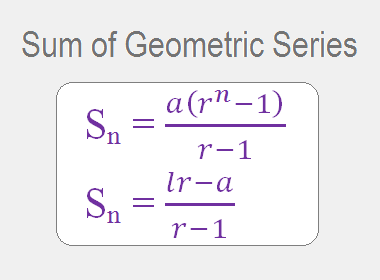The series associated with a geometric sequence is known as a geometric series. For example, 2 + 4 + 8 + 16 + 32 is the geometric series associated with the geometric sequence 2, 4, 8, 16, 32.

Let a be the first term, r be the common ratio, n be the number of terms, l be the last term and Sn be the sum to n terms of GS, then

Sn = a + ar + ar2 + … … … + arn-2 + arn-1 ………. (i)

Multiplying both sides by r,

rSn = ar + ar2 + ar3 + … … … + arn-1 + arn …….. (ii)

Subtracting (i) from (ii), we get

(r – 1)Sn = -a + arn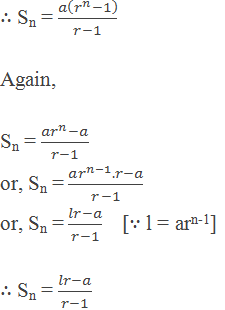## Worked Out Example

Example 1: Find the sum of the geometric series 1 + 2 + 4 + … … … 7 terms.

Solution:

Here,

First term (a) = 1

Common ratio (r) = 2/1 = 2

Number of terms (n) = 7

Sum of the terms (Sn) = ?

By using formula,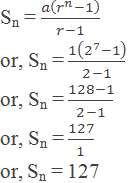Sn = 127

Example 2: In a GP, the first term is 7, the last term is 448 and the sum is 889, find the common ratio.

Solution:

Here,

First term (a) = 7

Last term (l) = 448

Sum of terms (Sn) = 889

Common ratio (r) = ?

By using the formula,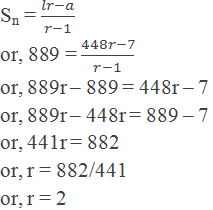The common ratio is 2.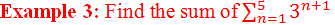Solution:

Here,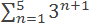= 31+1 + 32+1 + 33+1 + 34+1 + 35+1

= 32 + 33 + 34 + 35 + 36

= 9 + 27 + 81 + 243 + 729

= 1089

Example 4: How many terms of the series 32 + 48 + 72 + … … will add upto 665?

Solution:

Here,

First term (a) = 32

Common ratio (r) = 48/32 = 3/2

Sum of terms (Sn) = 665

Number of terms (n) = ?

By using formula,n = 6

The number of terms = 6

Example 5: If S3 and S6 of a GS are 7 and 63 respectively, find the common ratio.

Solution:

Here,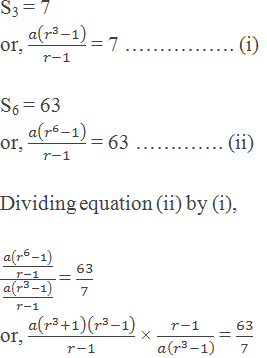or, r3 + 1 = 9

or, r3 = 9 – 1

or, r3 = 8

or, r3 = 23

or, r = 2

Common ratio = 2

Example 6: The second and fifth terms of GS are 3 and 81 respectively. Find the sum of the first five terms.

Solution:

Here,

The second term of GS is 3

i.e. t2 = 3

or, ar = 3

Again, the fifth term of GS is 81

i.e. t5 = 81

or, ar4 = 81

or, ar.r3 = 81

or, 3.r3 = 81

or, r3 = 81/3

or, r3 = 27 = 33

or, r = 3

And, ar = 3

or, a×3 = 3

or, a = 1

Now,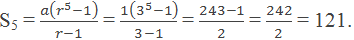Example 7: Find the GP for which the sum of the first two terms is -4 and the fifth term is 4 times the third term.

Solution:

Here,

S2 = -4

i.e. t1 + t2 = -4

or, a + ar = -4

or, a(1 + r) = -4 ……………… (i)

t5 = 4 × t3

i.e. ar4 = 4 × ar2

or, r2 = 4

or, r = ±2

Taking r = 2, from equation (i), we have

a(1 + 2) = -4

or, a = -4/3

The GP is a, ar, ar2, …

i.e. -4/3, -4×2/3, -4×22/3, …

i.e. -4/3, -8/3, -16/3, …

Taking r = -2, from equation (i), we have

a(1 – 2) = -4

or, a = 4

The GP is a, ar, ar2, …

i.e. 4, 4×-2, 4×(-2)2, …

i.e. 4, -8, 16, …

Example 8: If the sum of the first three terms of a GP is 1 and the sum of the first six terms is 28. Find the sum of the first 9 terms of the series.

Solution:

Here,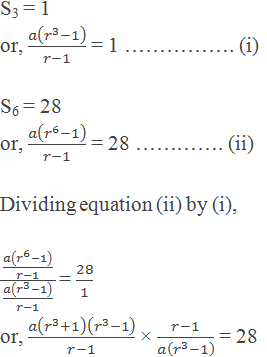or, r3 + 1 = 28

or, r3 = 28 – 1

or, r3 = 27

or, r3 = 33

or, r = 3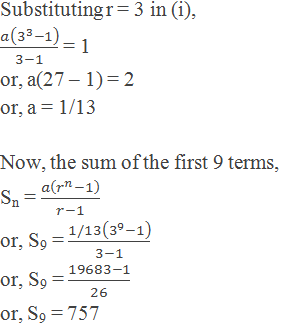The sum of first 9 terms is 757.